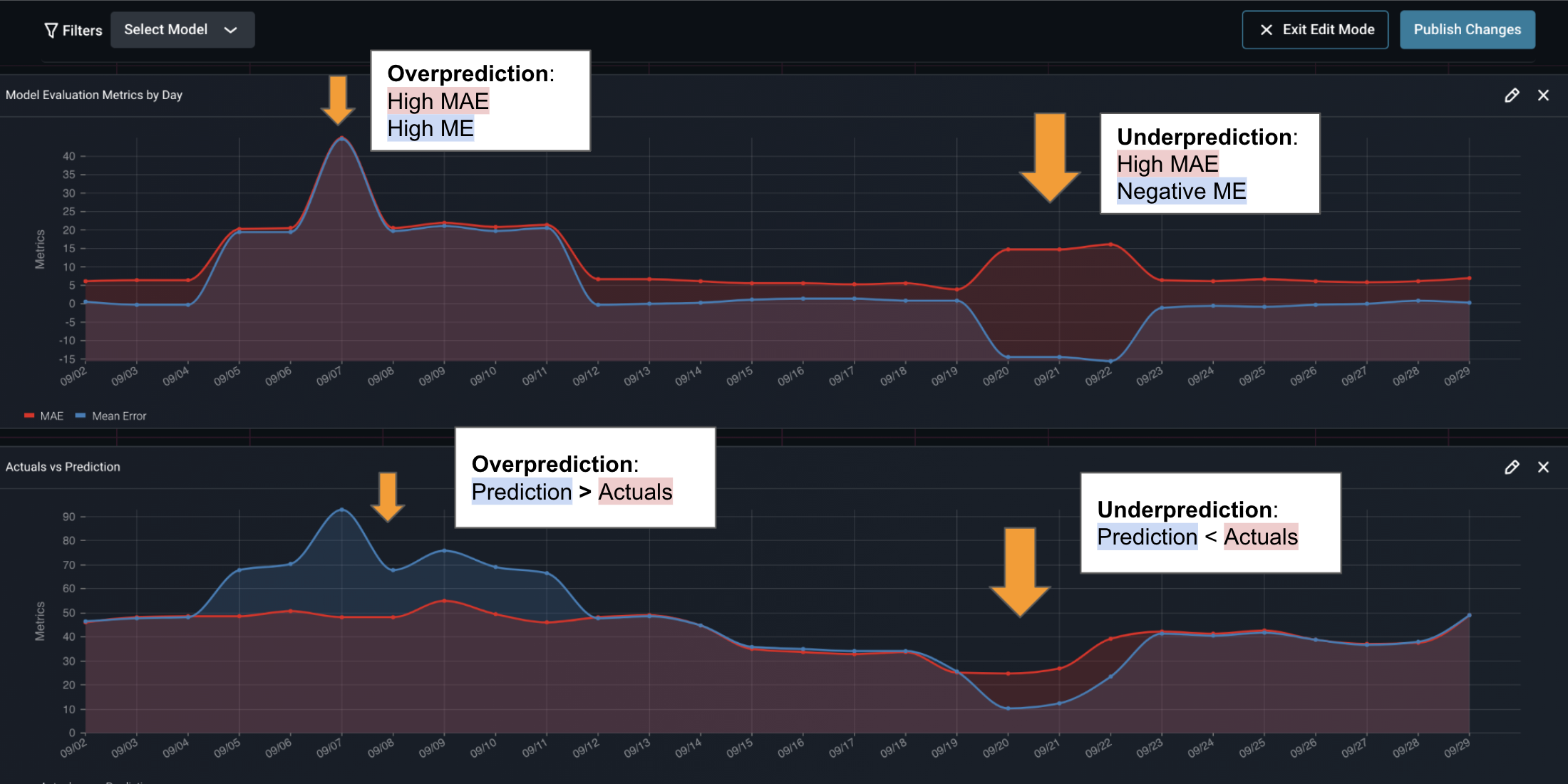# Timeseries Forecasting

Timeseries Prediction

### Overview

This example runs through how to setup a time series forecasting model in the Arize platform. In the timeseries forecasting use cases a model is run on specific run-date predicting data for a forecasting date in the future. This example will be predicting supply of a product from today, for each day, 10 days in the future.
This setup can be modified for predicting:
• End of quarter sales run every day until end of the quarter
• Predicting a single day in the future 100 days out - run every day
• Predicting sales of a product every day looking out over the next 30 daysExample of Timeseries Forecasting
The example above shows a supply model that makes 10 predictions for each day looking forward from the model run-date. The lag captures how many days ahead of the model run-date the specific prediction is for, where lag 0 is the actual day of the run.

### Common Observability Data for Timeseries Model

The common data that is tracked for timeseries models includes:
• Forecast Date: The date and time of the predicted event or observation.
• Run Date: The date on which your model ran and generated a prediction.
• Lag: The number of days between the run date and the forecast date.
For example, if you run a model on Monday to predict the temperature on Friday, the run date would be Monday's date, the forecast date would be a timestamp for a time on Friday and the lag would be four days.Setting up TimeSeries Data
The above picture shows how the data is mapped into the Arize platform. The timestamp is the forecast date, the run-date & lag is sent in as a tag and the actual prediction is sent in as the prediction label.

### Common Performance Metrics

The common metrics for timeseries forecasting are:
• MAE
• RMSE
• MSE
• MAPE
Filter options: Run-date, Lag
In the Arize Dashboard shown below, it's clear that we see an over prediction event first, then an under prediction event later. We can clearly see the magnitude of these errors based on our custom configuration.Timeseries MAPE
The above MAE shows predictions vs actuals for various forecast dates. In many scenarios teams want filtered by Lag < 10 looking at MAE for predictions only 10 days out.

### Example

The Colab example below shows how a timeseries model is setup in the Arize platform.
This Colab example shows how to configure a timeseries with multiple quantile forecasts in Arize, and how to configure Pinball Loss as a Custom Metric.